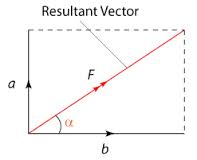2014-12-08

Resultant Force II

For the resultant force of two perpendicular forces, we need to consider other methods.

In this situation, two non-parallel forces are acting on an object at a right angle to each other.So with the example above, there is Force a (Sometimes called component a) and Force b (sometimes called component b).

The resultant force is named as F.

The resultant force can be obtained through Phytogras theorem

F^2 = a^2 + b^2

F = Square root of (a^2+b^2)Example of question:

What is the resultant force if Force a,70N  and Force b, 90N act on an object and both for the forces are perpendicular to each other. What is the direction of the force?

i. Resultant force, Fr = Square root of (70^2 + 90^2)
= 114N

ii. Direction, Tan (theta) = 70 / 90
= 0.7778
= 37.9 degree

So the resultant force is 114N and the direction is 37.9 degree from the original 90N force.

If you have known the basic principle.
The questions can be manipulated but you can still know how to work out the question.Ibphysics Tutions said...

Awesome blog and thanks for sharing
Physics Tuitions Bangalore

Ibphysics Tutions said...

Thank you very much for this great archive!
IB Standard Level Physics | IB Higher Level Physics

Mobile App Developers said...

Amazing blog and very interesting stuff you got here! I definitely learned a lot from reading through some of your earlier posts as well and decided to drop a comment on this one!

App Developers India said...

That is an extremely smartly written article. I will be sure to bookmark it and return to learn extra of your useful information. Thank you for the post. I will certainly return.